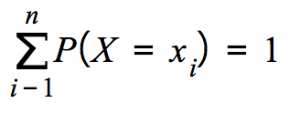# Basic Probability Formulas

0 ≤ P(A) ≤ 1

#### Rule of Complementary Events:

P(AC) + P(A) = 1

P(A∪B) = P(A) + P(B) – P(A∩B)

#### Disjoint Events:

Events A and B are disjoint iff
P(A∩B) = 0

#### Conditional Probability:

P(A | B) = P(A∩B) / P(B)

#### Bayes Formula:

P(A | B) = P(B | A) ⋅ P(A) / P(B)

#### Independent Events:

Events A and B are independent iff
P(A∩B) = P(A) ⋅ P(B)

FX(x) = P(X ≤ x)

FX(x) = P(X ≤ x)

#### Probability Mass Function:#### Probability Density Function:#### Covariance:#### Correlation:Bernoulli: 0-failure 1-success

Geometric: 0-failure 1-success

Hypergeometric: N objects with K success objects, n objects are taken.